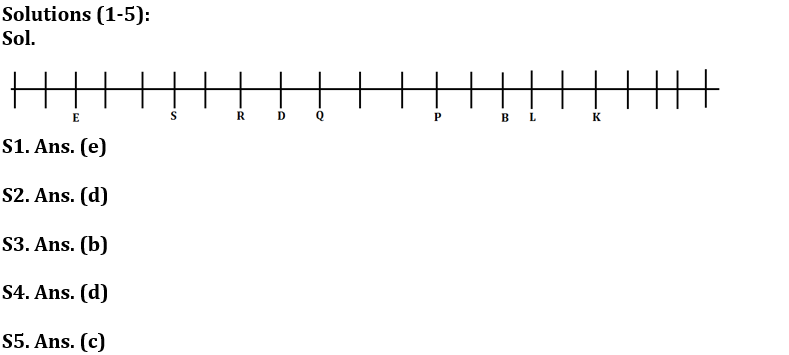Latest Banking jobs   »   Reasoning Ability Quiz For FCI Phase...

# Reasoning Ability Quiz For FCI Phase I 2023- 5th January

Directions (1-5): Study the information carefully and answer the questions given below.
A certain number of persons sit in a row. All of them are facing north. P sits third to the right of Q. Four persons sit between R and P. S sits second to the left of R. Four persons sits between R and E. E sits third from one of the extreme ends. B sits sixth to the right of D who is an immediate neighbor of R. As many persons between E and D as many between Q and L. L does not sit left of Q. K is fifth from one of the extreme ends and one person sit between L and K. Q does not sit left of D. K is not an immediate neighbour of P.

Q1. How many persons sit in row?
(a) 18
(b) 19
(c) 20
(d) 21
(e) None of these

Q2. How many persons sit between B and S?
(a) 7
(b) 9
(c) 10
(d) 8
(e) None of these

Q3. Who among the following person sit third to the left of L?
(a) Q
(b) P
(c) B
(d) D
(e) No one

Q4. Four of the following five are alike in certain way based from a group, find the one that does not belong to that group?
(a) Q-P
(b) D-S
(c) B-K
(d) K-P
(e) L-P

Q5. How many persons sit left of D?
(a) Nine
(b) Four
(c) Eight
(d) Seven
(e) None of these

Directions (6-10): Following questions are based on the five three-digit numbers given below.

947 376 863 694 739

Q6. If all the digits in each of the numbers are arranged in increasing order within the number, then, which of the following number will become the lowest in the new arrangement of numbers?
(a) 947
(b) 863
(c) 739
(d) 694
(e) 376

Q7. If all the numbers are arranged in ascending order from left to right then, which of the following will be the sum of all the three digits of the number which is 2nd from the right in the new arrangement?
(a) 18
(b) 19
(c) 15
(d) 16
(e) None of these

Q8. What will be the difference, when third digit of the 3rd lowest number is multiplied with the second digit of the highest number and third digit of the 2nd highest number is multiplied with the second digit of the lowest number?
(a) 21
(b) 20
(c) 15
(d)16
(e) None of these

Q9. If the positions of the second and the third digits of each of the numbers are interchanged then, how many even numbers will be formed?
(a) None
(b) One
(c) Two
(d) Three
(e) Four

Q10. If one is added to the second digit of each of the numbers and one is subtracted to the third digit of each number then, how many numbers thus formed will be divisible by three in new arrangement?
(a) None
(b) One
(c) Two
(d) Three
(e) Four

Q11. If all the letters in the word PAINTBALL are arranged in alphabetical order from left to right in such a way that vowels are arranged first followed by consonants, then how many letters are there in between I and N after the arrangement?
(a) Two
(b) Three
(c) None
(d) One
(e) Four

Q12. If in the number 82164739, 1 is added to each of the digit which is less than five and 1 is subtracted from each of the digit which is greater than five then how many digits are repeating in the number thus formed?
(a) One
(b) Two
(c) None
(d) Three
(e) Four

Q13. The position of how many alphabets will remain unchanged if each of the alphabets in the word ‘PARANORMAL’ is arranged in alphabetical order from left to right?
(a) Two
(b) One
(c) None
(d) Three
(e) Four

Q14. Which of the following elements should come in a place ‘?’ ?
JK12 LM14 NO16 PQ18 ?
(a) ST21
(b) RS20
(c) TU22
(d) UV23
(e) None of these

Q15. Sumit is 18th from the left end of a row and Adarsh is 10th from the right end of row. If they interchanged their positions then adarsh ranks become 22 from right end. Find total number of persons in the row?
(a) 40
(b) 39
(c) 38
(d) 41
(e) None of these

SolutionsS6. Ans. (e)

S7. Ans. (e)

S8. Ans. (c)

S9. Ans. (c)

S10. Ans. (a)

S11. Ans. (b)

S12. Ans. (a)

S13. Ans. (b)

S14. Ans. (b)

S15. Ans. (b)
Sol. Total number of persons in the row= (18+22-1) = 39## FAQs

#### Congratulations!